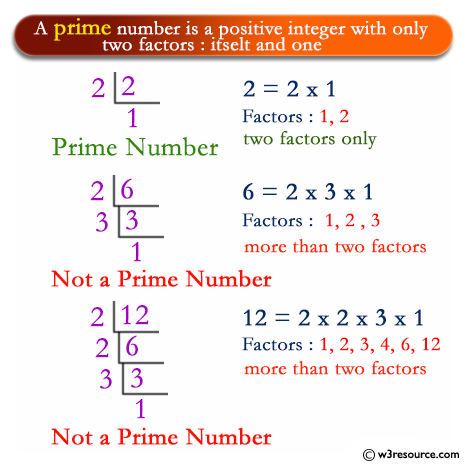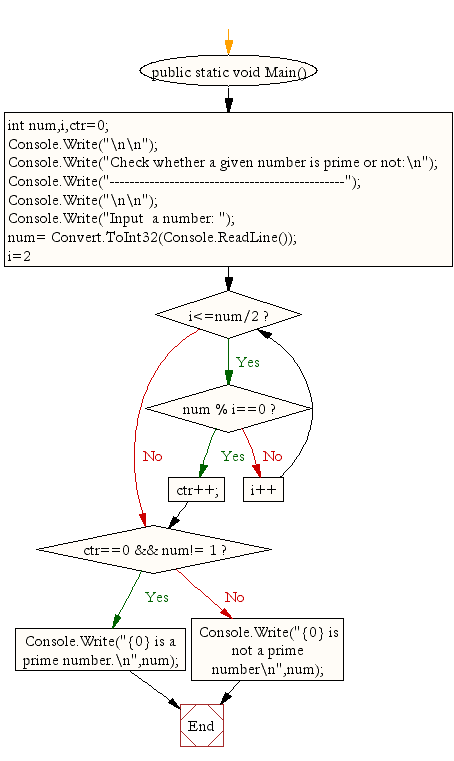﻿ C# - Check whether a given number is prime or not# C# Sharp Exercises: Check whether a given number is prime or not

## C# Sharp For Loop: Exercise-32 with Solution

Write a C# Sharp Program to determine whether a given number is prime or not.

Pictorial Presentation:Sample Solution:

C# Sharp Code:

``````using System;
public class Exercise32
{
public static void Main()
{

int num,i,ctr=0;

Console.Write("\n\n");
Console.Write("Check whether a given number is prime or not:\n");
Console.Write("-----------------------------------------------");
Console.Write("\n\n");

Console.Write("Input  a number: ");
for(i=2;i<=num/2;i++){
if(num % i==0){
ctr++;
break;
}
}
if(ctr==0 && num!= 1)
Console.Write("{0} is a prime number.\n",num);
else
Console.Write("{0} is not a prime number\n",num);
}
}
```
```

Sample Output:

```Check whether a given number is prime or not:
-----------------------------------------------
Input  a number: 53
53 is a prime number.
```

Flowchart:C# Sharp Code Editor:

Contribute your code and comments through Disqus.

What is the difficulty level of this exercise?

Test your Programming skills with w3resource's quiz.

﻿